CBSE Class 10 Sample Paper for 2021 Boards - Maths Basic

Class 10
Solutions of Sample Papers for Class 10 Boards

## Rahul is studying in X Standard. He is making a kite to fly it on a Sunday. Few questions came to his mind while making the kite. Give answers to his questions by looking at the figure

Note: Check more Case Based Questions for - Triangles Class 10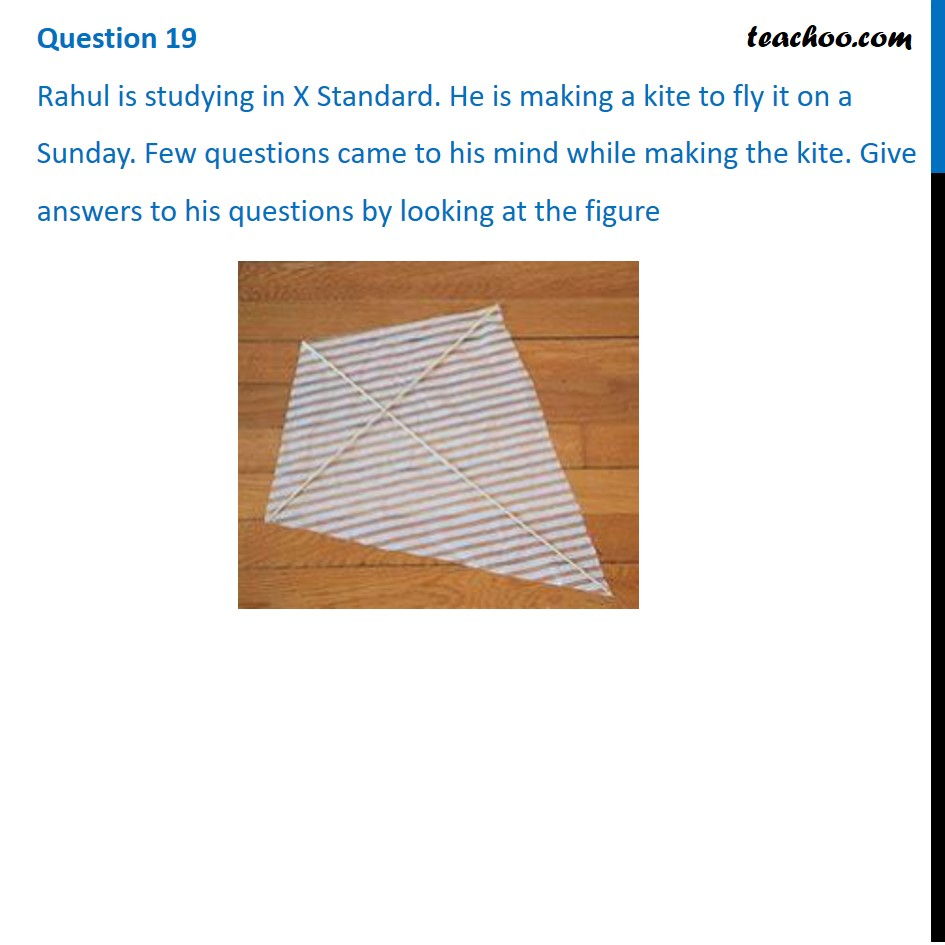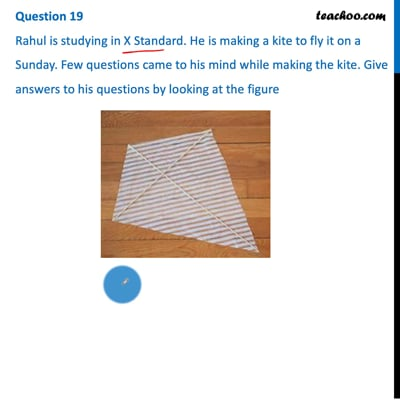This video is only available for Teachoo black users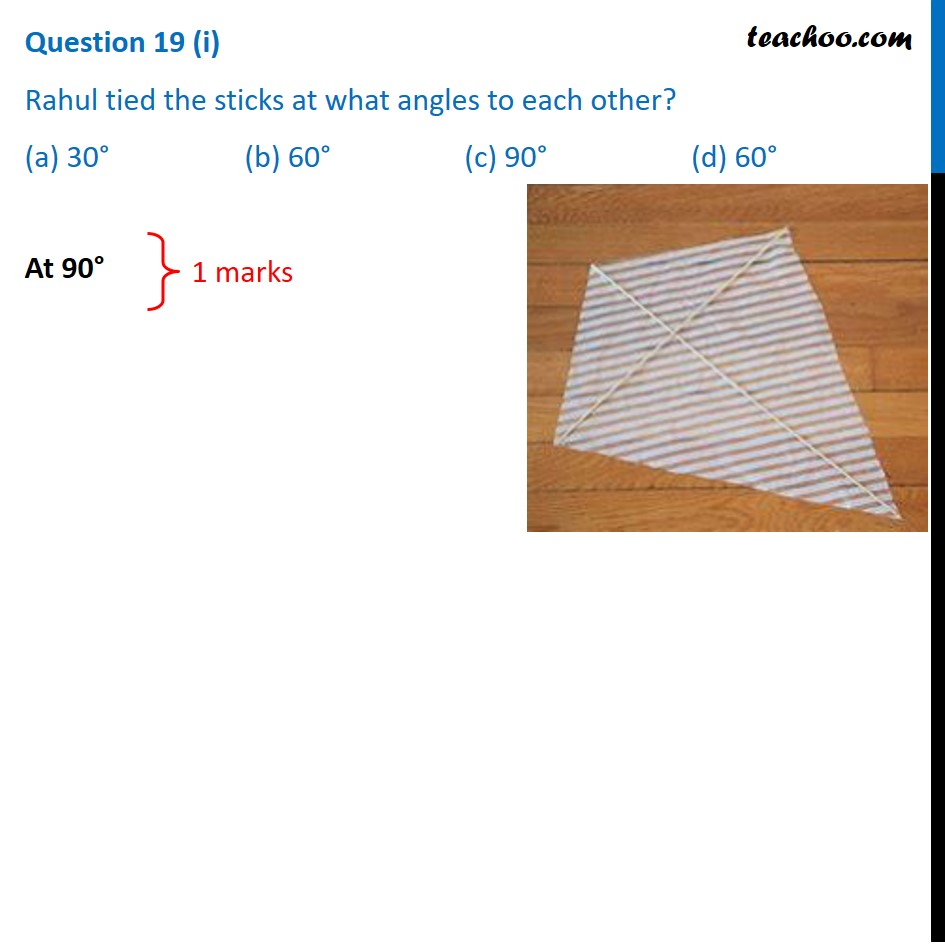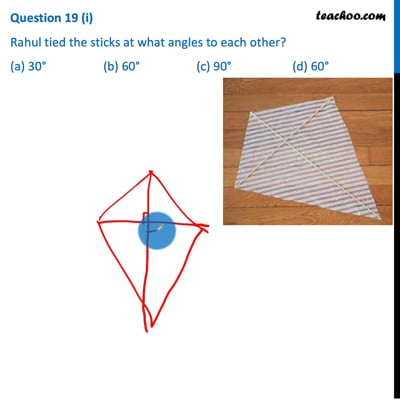This video is only available for Teachoo black users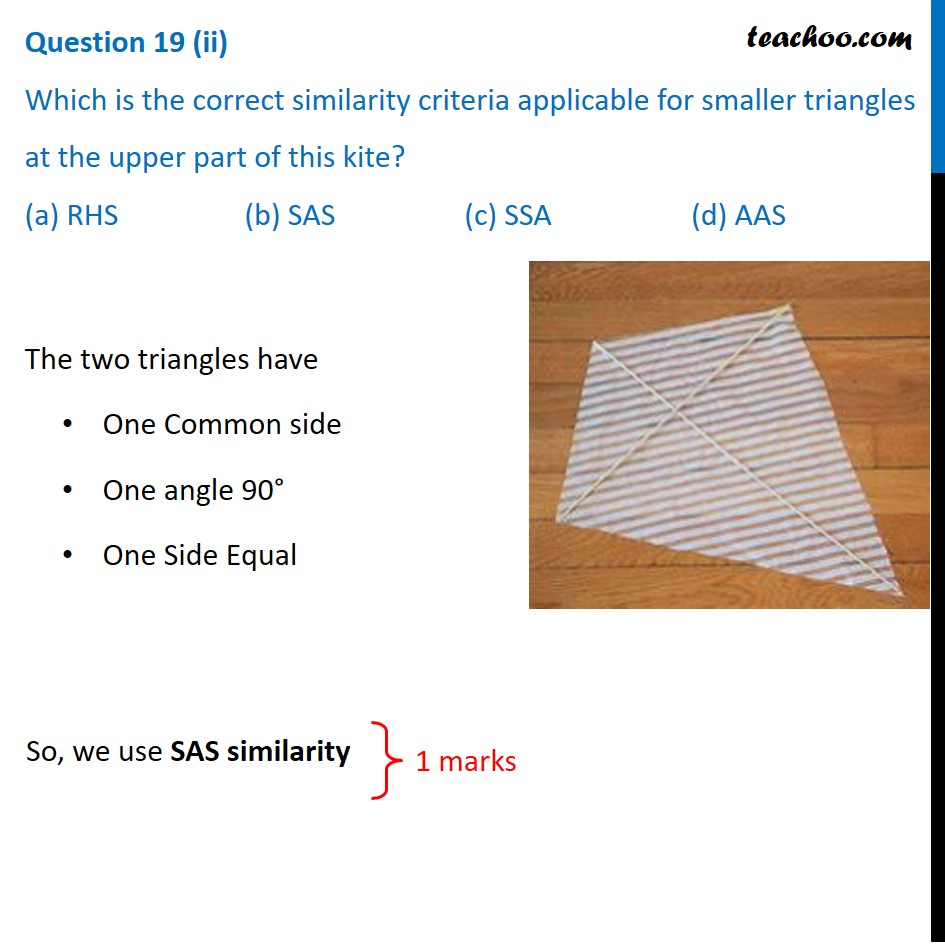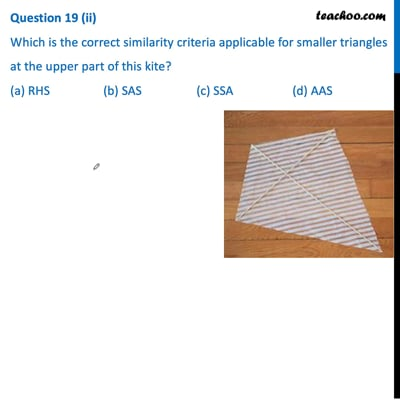This video is only available for Teachoo black users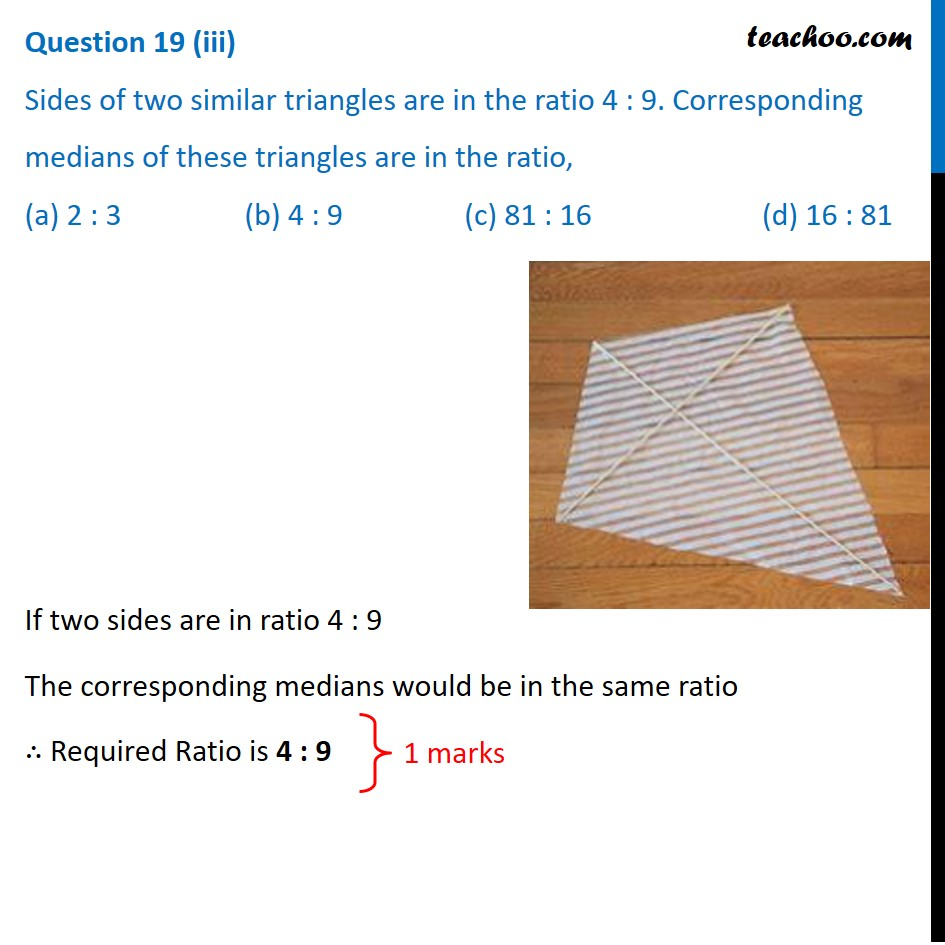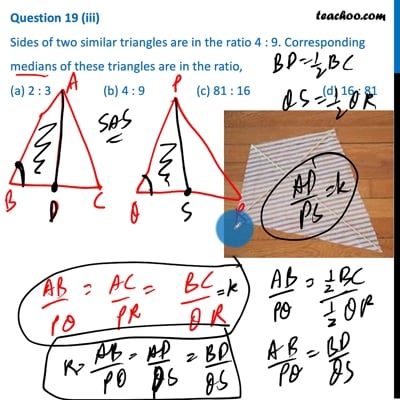This video is only available for Teachoo black users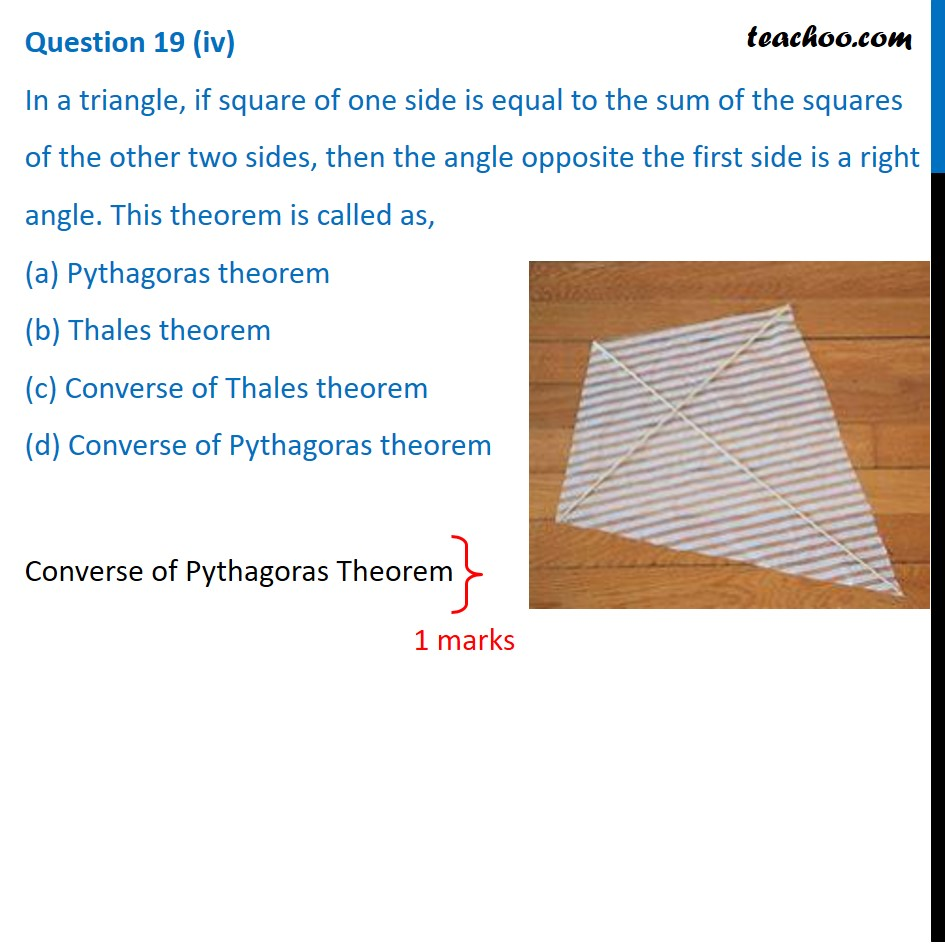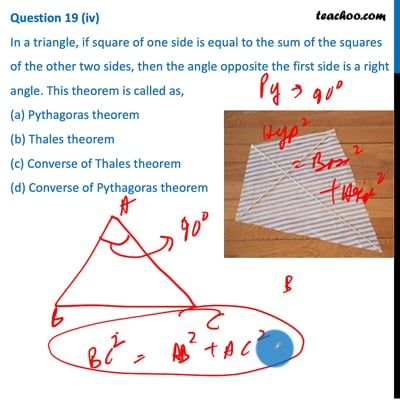This video is only available for Teachoo black users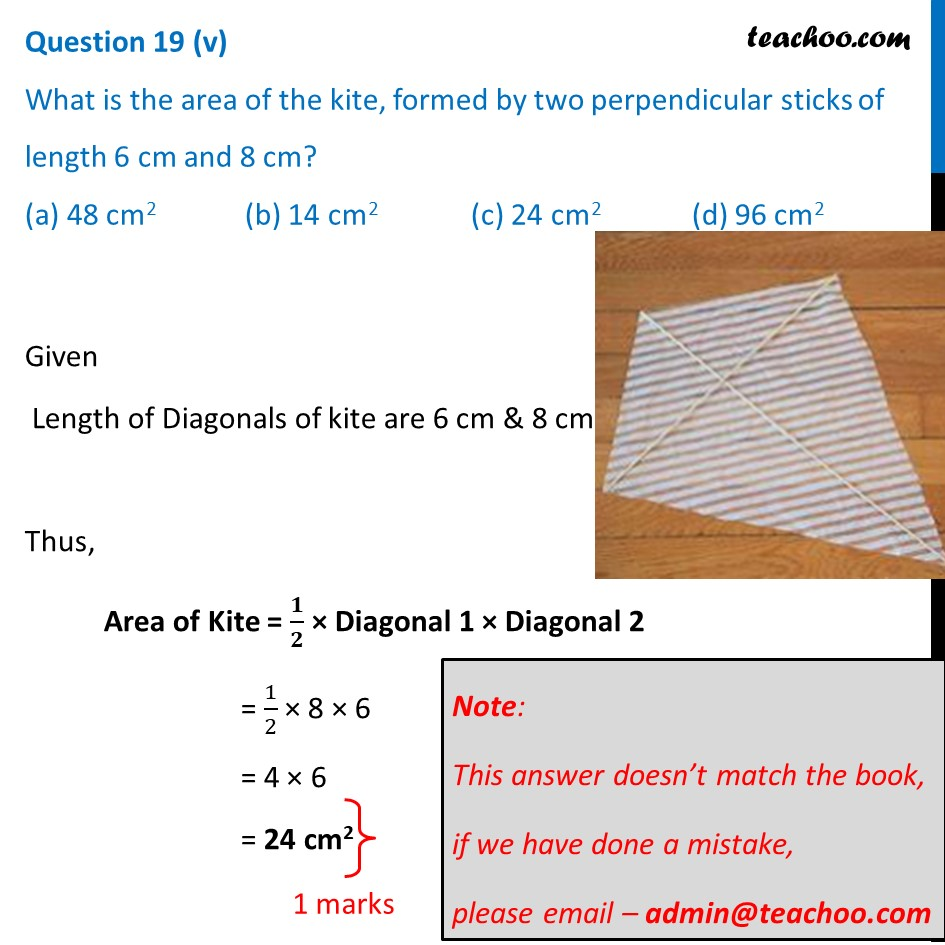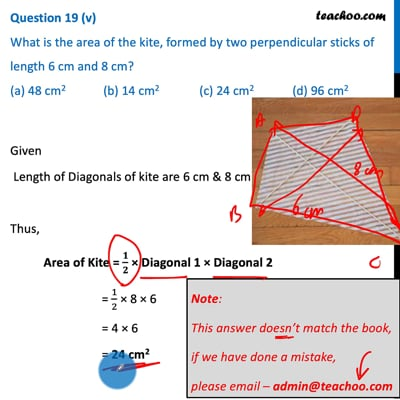This video is only available for Teachoo black users

Note: Check more Case Based Questions for - Triangles Class 10

Introducing your new favourite teacher - Teachoo Black, at only ₹83 per month

### Transcript

Question 19 Rahul is studying in X Standard. He is making a kite to fly it on a Sunday. Few questions came to his mind while making the kite. Give answers to his questions by looking at the figureQuestion 19 (i) Rahul tied the sticks at what angles to each other? (a) 30° (b) 60° (c) 90° (d) 60° At 90° Question 19 (ii) Which is the correct similarity criteria applicable for smaller triangles at the upper part of this kite? (a) RHS (b) SAS (c) SSA (d) AAS The two triangles have One Common side One angle 90° One Side Equal So, we use SAS similarity Question 19 (iii) Sides of two similar triangles are in the ratio 4 : 9. Corresponding medians of these triangles are in the ratio, (a) 2 : 3 (b) 4 : 9 (c) 81 : 16 (d) 16 : 81 If two sides are in ratio 4 : 9 The corresponding medians would be in the same ratio ∴ Required Ratio is 4 : 9 1 marks Question 19 (iv) In a triangle, if square of one side is equal to the sum of the squares of the other two sides, then the angle opposite the first side is a right angle. This theorem is called as, (a) Pythagoras theorem (b) Thales theorem (c) Converse of Thales theorem (d) Converse of Pythagoras theorem Converse of Pythagoras Theorem 1 marks Question 19 (v) What is the area of the kite, formed by two perpendicular sticks of length 6 cm and 8 cm? (a) 48 cm2 (b) 14 cm2 (c) 24 cm2 (d) 96 cm2 Given Given Length of Diagonals of kite are 6 cm & 8 cm Thus, Area of Kite = 𝟏/𝟐 × Diagonal 1 × Diagonal 2 = 1/2 × 8 × 6 = 4 × 6 = 24 cm2